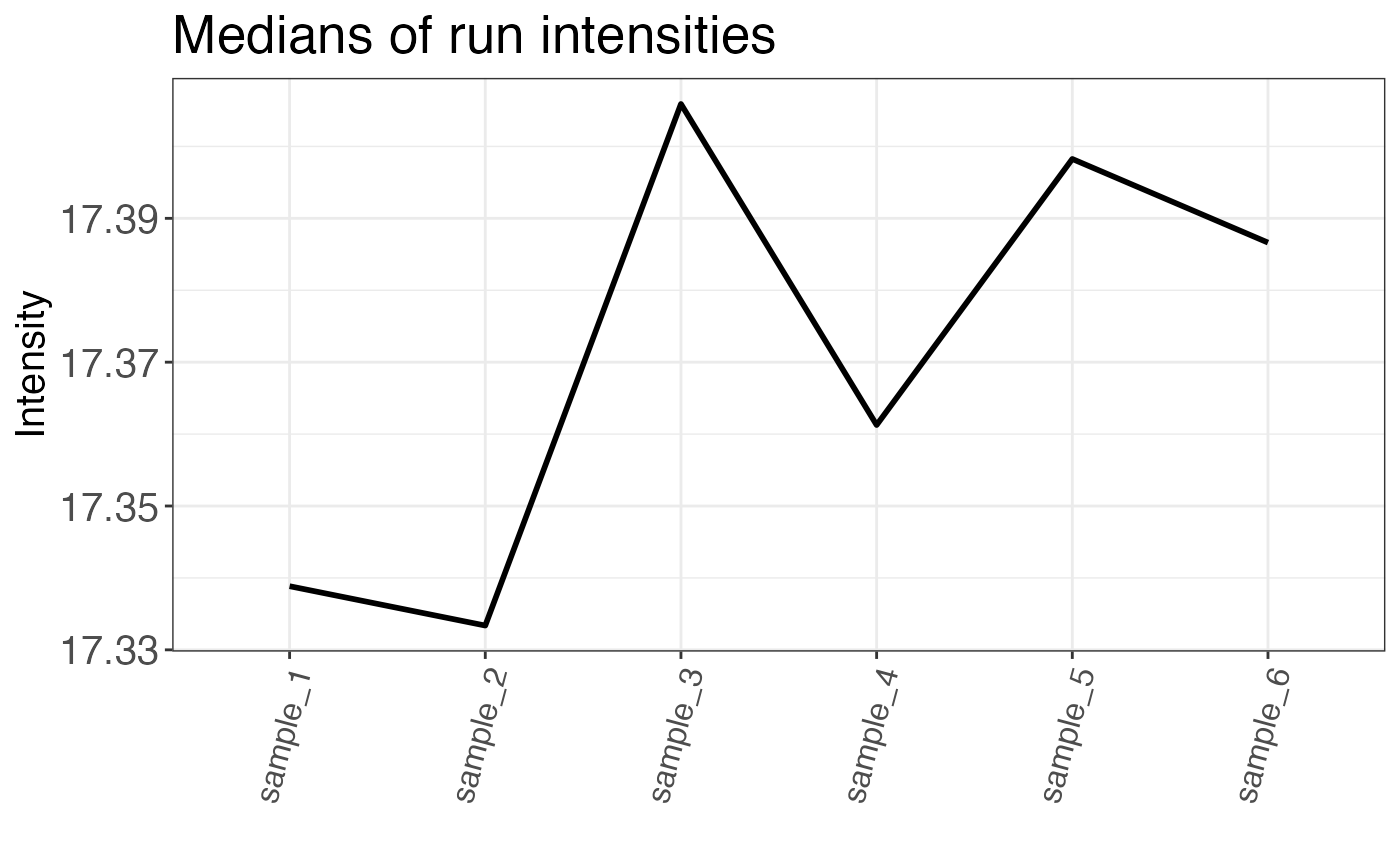Median intensities per run are returned either as a plot or a table.

qc_median_intensities(
data,
sample,
grouping,
intensity,
plot = TRUE,
interactive = FALSE
)

## Arguments

data a data frame that contains at least the input variables. a character column in the data data frame that contains the sample name. a character column in the data data frame that contains either precursor or peptide identifiers. a numeric column in the data data frame that contains intensity values. The intensity should be ideally log2 transformed, but also non-transformed values can be used. a logical value that indicates whether the result should be plotted. a logical value that specifies whether the plot should be interactive (default is FALSE).

## Value

A plot that displays median intensity over all samples. If plot = FALSE a data frame containing median intensities is returned.

## Examples

set.seed(123) # Makes example reproducible

# Create example data
data <- create_synthetic_data(
n_proteins = 100,
frac_change = 0.05,
n_replicates = 3,
n_conditions = 2,
method = "effect_random"
)

# Calculate median intensities
qc_median_intensities(
data = data,
sample = sample,
grouping = peptide,
intensity = peptide_intensity_missing,
plot = FALSE
)
#> # A tibble: 6 × 2
#>   sample   median_intensity
#>   <chr>               <dbl>
#> 1 sample_1             17.3
#> 2 sample_2             17.3
#> 3 sample_3             17.4
#> 4 sample_4             17.4
#> 5 sample_5             17.4
#> 6 sample_6             17.4
# Plot median intensities
qc_median_intensities(
data = data,
sample = sample,
grouping = peptide,
intensity = peptide_intensity_missing,
plot = TRUE
)